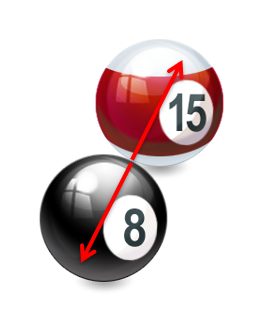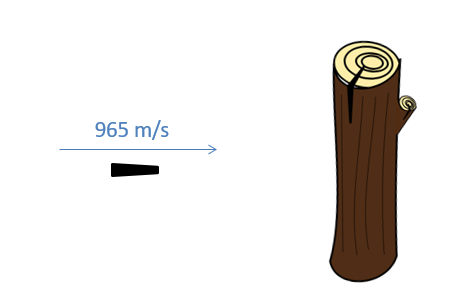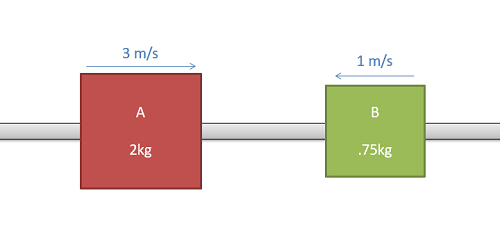﻿ Mechanics Map - Particle Collisions in One Dimension

# Particle Collisions in One Dimension

The Impulse-Momentum Method is particularly useful when examining collisions between bodies. When examining the bodies colliding as a system of particles, such as the two bodies colliding below, the impulses exerted by internal forces, that is the forces exerted between two bodies within the system, will be equal and opposite and hence cancel out in our impulse momentum equation.When two bodies collide, Newton's Third Law ensures that the normal forces from the collision will always be equal and opposite. This means that the impulses will be equal and opposite and will cancel out in our impulse-momentum equation when examining the system of equations.

Because the impulsive forces of the collision are so large over the very short period of the collision, other external forces are typically regarded as insignificant. This means that for our collision, there is no external impulse and therefore there is no change to the sum of the momentums of the bodies. This is known as the conservation of momentum, and it will hold true for all types of collisions.

 $\sum \vec{J} = 0 =\sum m \vec{v_{f}}-\sum m \vec{v_{i}}$ $\sum m \vec{v_{f}}=\sum m \vec{v_{i}}$

In the case of the collision of two bodies, body A and body B in this case, we can break apart the sums as follows. The subscripts in this case are being used to denote both the body (A or B) and initial or final states (before or after the collision).

 $m_{A} \vec{v_{Af}}+m_{B} \vec{v_{Bf}}= m_{A} \vec{v_{Ai}}+m_{B} \vec{v_{Bi}}$

In addition to the conservation of momentum equation, we will also usually generate a second equation we can use alongside the conservation of momentum. This second equation will depend on the type of collision though, with the three possible collision types being elastic, inelastic, and semi-elastic.

## Elastic Collisions:

An elastic collision is a collision in which all energy is assumed to be conserved as kinetic energy. In reality, true elastic collisions do not exist as some energy will always be converted to heat or sound, but in practice two very rigid bodies that collide without much deformation can get very close to the ideal of an elastic collision. An example of a collision that is close to elastic is a set of billiard balls colliding, as in the earlier figure.

An elastic collision conserves energy in addition to momentum, so the conservation of energy equation will be our second equation that we use to supplement the conservation of momentum.

 $\frac{1}{2}m_{A} \vec{v_{Af}}^{2}+\frac{1}{2}m_{B} \vec{v_{Bf}}^{2}= \frac{1}{2}m_{A} \vec{v_{Ai}}^{2}+\frac{1}{2}m_{B} \vec{v_{Bi}}^{2}$

## Inelastic Collisions:

An inelastic collision is the opposite of an elastic collision in that much of the energy of the system is lost in the deformation of the bodies. In fact in an inelastic collision, the bodies must deform in such as way that they stick together after the collision. With the two bodies stuck together, they will have matching final velocities.

 $\vec{v_{Af}}=\vec{v_{Bf}}$

## Semi-Elastic Collisions:

Finally, anything between an elastic collision and an inelastic collision is considered a semi-elastic collision. This is a collision where less than one hundred percent of the kinetic energy is conserved, but the objects do not stick together following the collision. In cases of semi-elastic collisions, we will use something called the coefficient of restitution (usually denoted by Greek letter epsilon), to supplement our conservation of momentum equation.

The coefficient of restitution is number between 0 and 1 that measures the "bounciness" of the two bodies in the collision. Specifically, for a single body bouncing off a rigid surface, the coefficient of restitution is defined as the negative of the velocity after bouncing off a surface divided by the velocity before bouncing off the surface.

 $e=-\frac{\vec{v_{f}}}{\vec{v_{i}}}$

A perfectly elastic collision would have a coefficient of restitution of one (no velocity would be lost) while a plastic collision would have a coefficient of restitution of zero. All other collisions will have a coefficient of restitution that lies somewhere in between.

For cases where two bodies are bouncing off of one another, we would simply use relative velocities rather than the velocity of a single body. Using the velocity of body A relative to the velocity of body B, we come up with the equation below.

 $e=-\frac{\vec{v_{fA/B}}}{\vec{v_{iA/B}}}=-\frac{\vec{v_{Af}}-\vec{v_{Bf}}}{\vec{v_{Ai}}-\vec{v_{Bi}}}$

## Worked Problems:

### Question 1:

A cue ball weighing .17kg is traveling 1 m/s impacts a stationary billiard ball with a mass of .15kg as shown below. If the balls collide directly and the collision is elastic, what will the velocities be after the collision (ignore rotational energies)?### Question 2:

A 4.2g bullet traveling at 965 m/s becomes lodged in a stationary log with a mass of 1.5kg.

• What is the the velocity of the log and the bullet immediately after the collision?
• What percentage of the kinetic energy was lost in the collision?### Question 3:

Two masses on a frictionless rod as shown below are set to impact with each other. If the coefficient of restitution between the objects is .6, what is the velocity of each body after the collision?